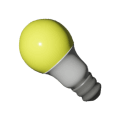Light bulb electrical usage | BCHydro Power Smart for Schools
Electricity

# The number of light bulbs in a home and electricity usage

Practice your mathematical thinking and develop your understanding of statistics while deciding what the number of light bulbs in a home might reveal.11Duration
20 minsType
Class discussion

## Overview

Using primary data about the number of light bulbs in their homes, students will calculate and interpret z-scores. Students will decide what this mathematical evidence might reveal about their home’s energy usage.

### Instructions

#### What you'll need

• "Analyzing the z-score" worksheet
• "Light bulb inventory" worksheet

1. Provide each of your students with a copy of the "Analyzing the z-score" worksheet. Invite students to record their initial thinking on how the number of lights in their household might compare to the classroom average. Encourage students to share their decisions and thinking with the class.
2. Invite your students to suggest what might be inferred about a household’s electricity usage by examining the number of lights in the home.
3. If your students have not yet completed the "How does your use of lights measure up?" activity, provide each student with the "Light bulb inventory" worksheet and ask them to do a mental walkthrough of their homes, recording the number of light bulbs in their home.
4. Ask students to complete the table and calculations on the worksheet to gather mathematical evidence.
5. Encourage students to revisit their initial conjecture, this time thinking about their z-scores. Prompt students to compare their initial conjecture with this new conclusion and to share their thinking with the class.
6. Invite students to suggest what might be inferred about a household’s electricity usage by examining the z-scores.

### Modify or extend this activity

#### Modifications

• Students may be invited to use a spreadsheet or similar technology to perform calculations.

#### Extensions

• Students may be invited to use a standard normal distribution table to determine what percentage (or number) of students use a number of lights higher and lower than themselves.
• Have students collect data for the amount of time each light bulb in their household is on during a typical day to determine the average amount of energy used by each light bulb and how their household lighting energy use compares to the average in their classroom community.

### Curriculum Fit

#### Content

• Applications of statistics
• Variation occurs in observation
• How do we analyze data and make decisions?
• How would you describe variation?
• When analyzing data, what are some factors that need to be considered before making an inference?
• Posing a question about an observed variation, collecting and interpreting data, to answer the question
• Measures of central tendency, standard deviation, z-scores and distribution

#### Curricular competencies

##### Reasoning and modelling
• Explore, analyze, and apply mathematical ideas using reason, technology, and other tools
##### Understanding and solving
• Visualize to create and use mental images to support understanding
• Develop, demonstrate and apply mathematical understanding through inquiry and problem-solving
##### Connecting and reflecting
• Reflect on mathematical thinking
• Connect mathematical concepts with each other, other areas, and personal interests
• Use mistakes as opportunities to advance learning

### Assessment

Throughout the activity consider how well students:

• Understand the statistical concept of variance by using and interpreting the statistical measure of z-scores
• Connect personal experiences within a broader context (the community)
• Use appropriate vocabulary (mean, standard deviation and z-score) in their justifications and/or explanations
• Pay close attention to appropriate details
• Use mathematical evidence to make sound mathematical decisions or conclusions